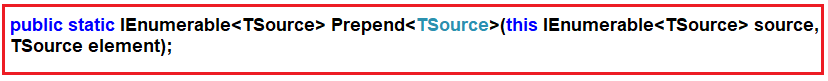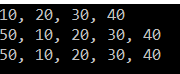# Linq Prepend Method in C#

## Linq Prepend Method in C# With an Example

In this article, I am going to discuss the LINQ Prepend Method in C# with an example. Please read our previous article before proceeding to this article where we discussed the LINQ Append Method with an example.

##### Linq Prepend Method in C#:

The Linq Prepend Method is used to add one value to the beginning of a sequence. This Prepend method like the Append method does not modify the elements of the sequence. Instead, it creates a copy of the sequence with the new element. The signature of this is given below.##### Type Parameters
1. TSource: The data type of the elements contained in the sequence.

Parameters:

1. IEnumerable<TSource> source: A sequence of values.
2. TSource element: The value to prepend at the beginning of the sequence.

Returns:

1. IEnumerable<TSource>: A new sequence that begins with the element.

Exceptions: When the source is null, it will throw ArgumentNullException.

Note: This method is support from Framework 4.7.1 or later.

##### Example:

The following example shows how to prepend a value to the beginning of the sequence using the Prepend method. The following example code is self-explained. So, please go through the comment lines.

```using System.Linq;
using System.Collections.Generic;
using System;
namespace LinqDemo
{
class Program
{
static void Main(string[] args)
{
// Creating a list of numbers
List<int> numberSequence = new List<int> { 10, 20, 30, 40 };

// Trying to prepend 50
numberSequence.Prepend(50);

// It will not work because the original sequence has not been changed
Console.WriteLine(string.Join(", ", numberSequence));

// It works now because we are using a changed copy of the original list
Console.WriteLine(string.Join(", ", numberSequence.Prepend(50)));

// If you prefer, you can create a new list explicitly
List<int> newnumberSequence = numberSequence.Prepend(50).ToList();

// And then write to the console output
Console.WriteLine(string.Join(", ", newnumberSequence));